Ex 6.5

Chapter 6 Class 12 Application of Derivatives (Term 1)
Serial order wise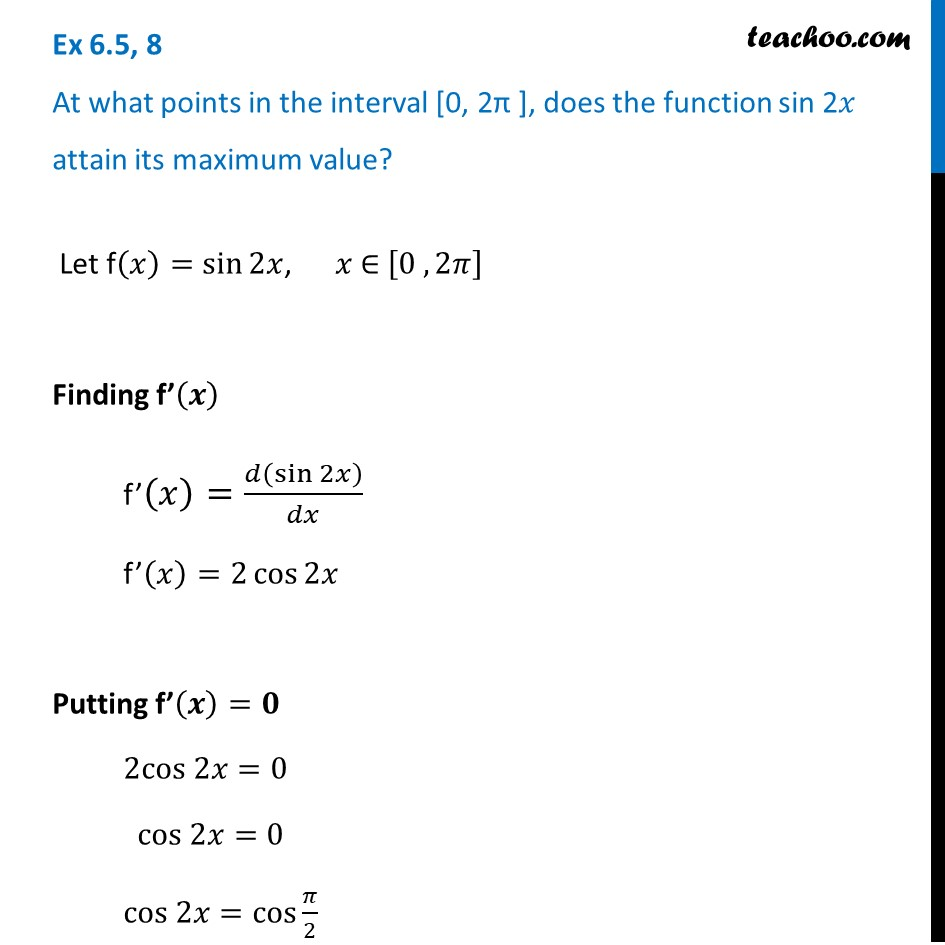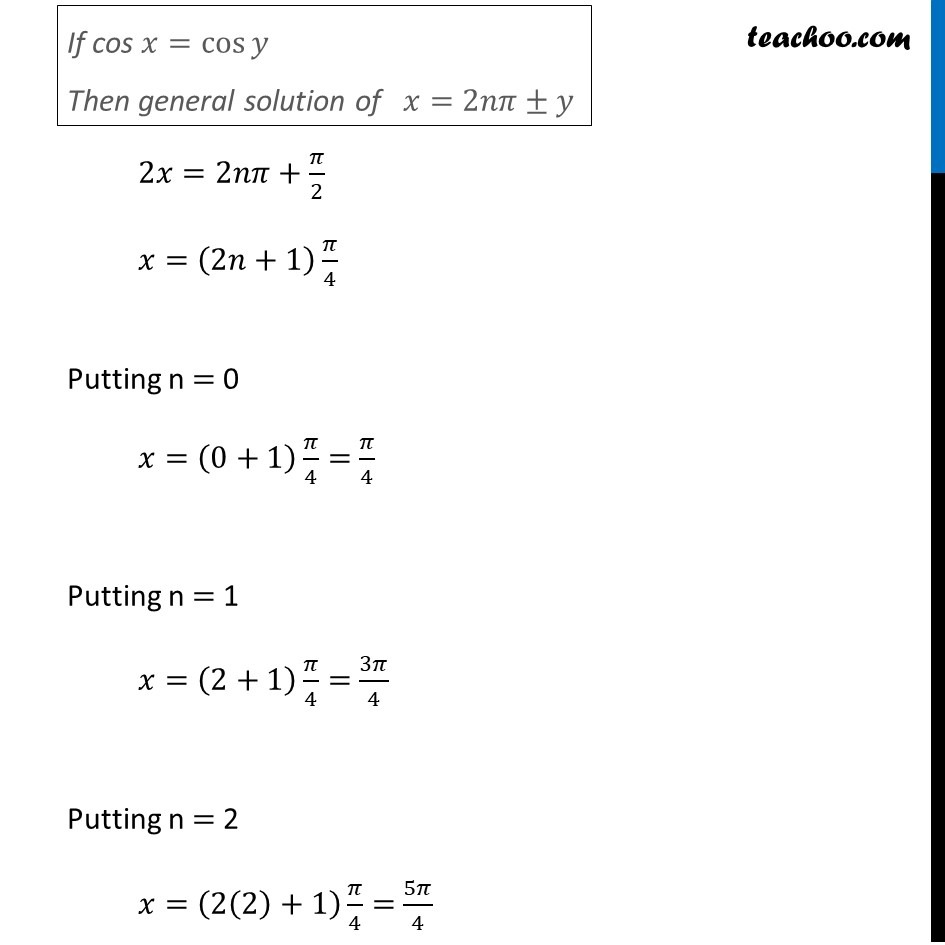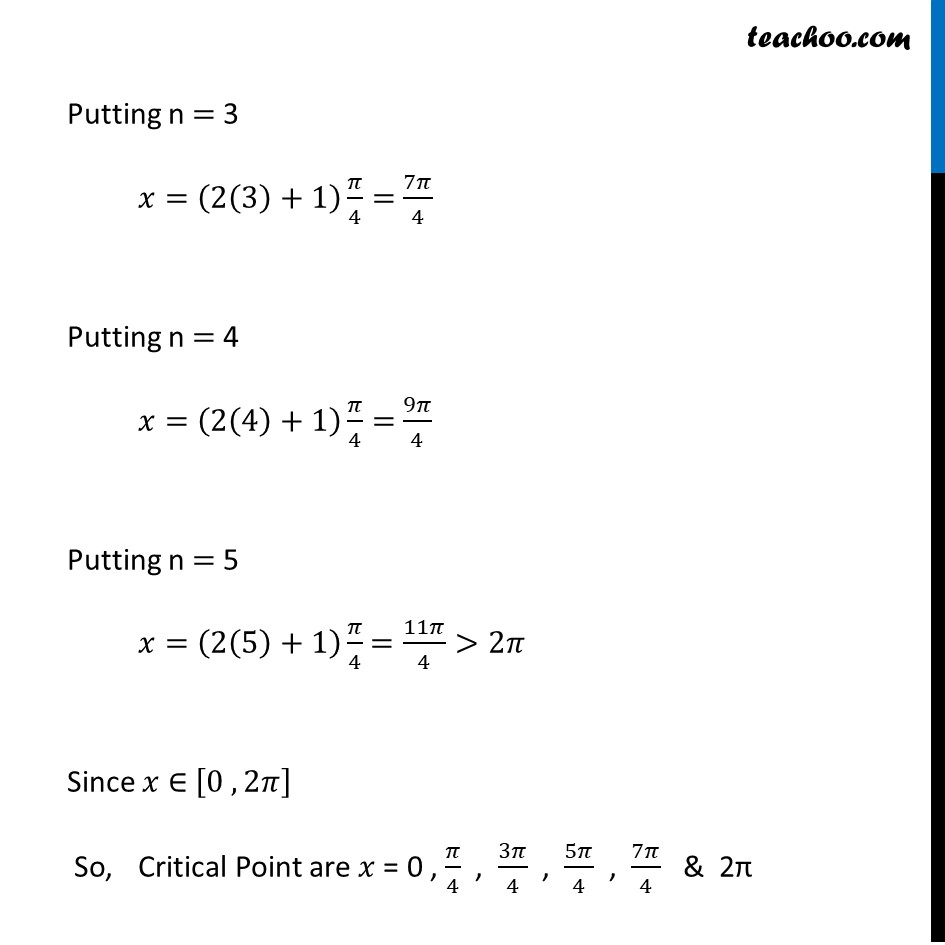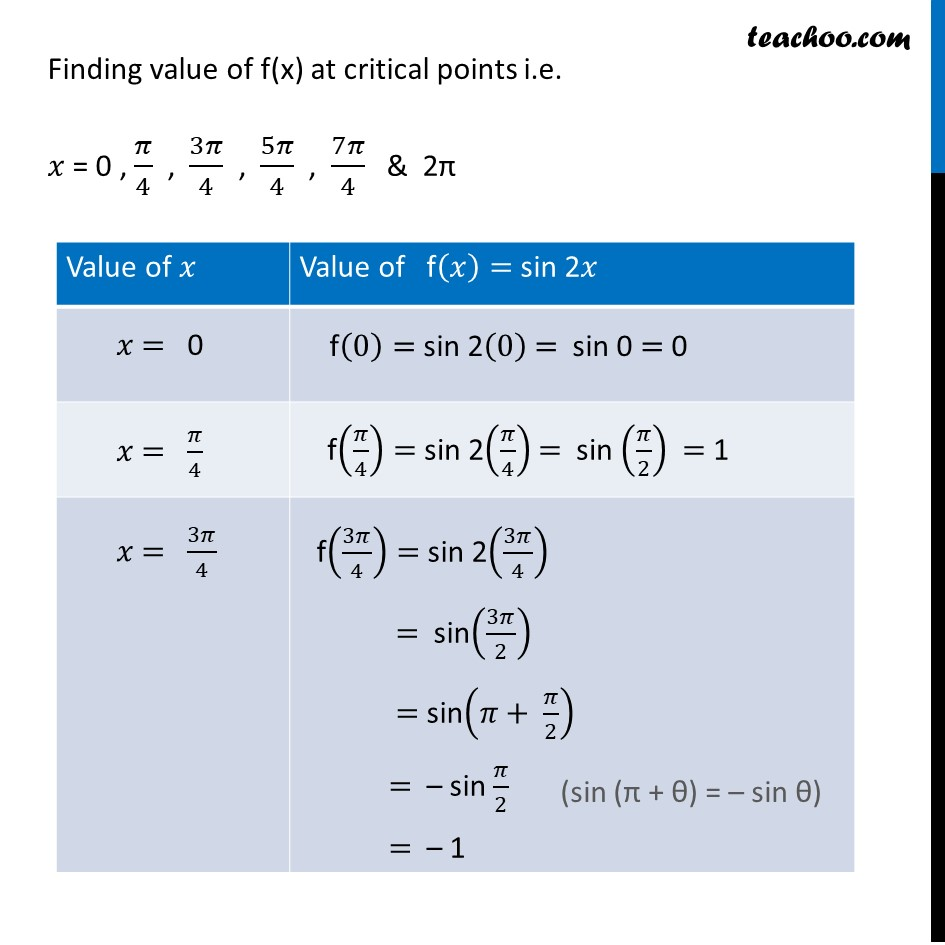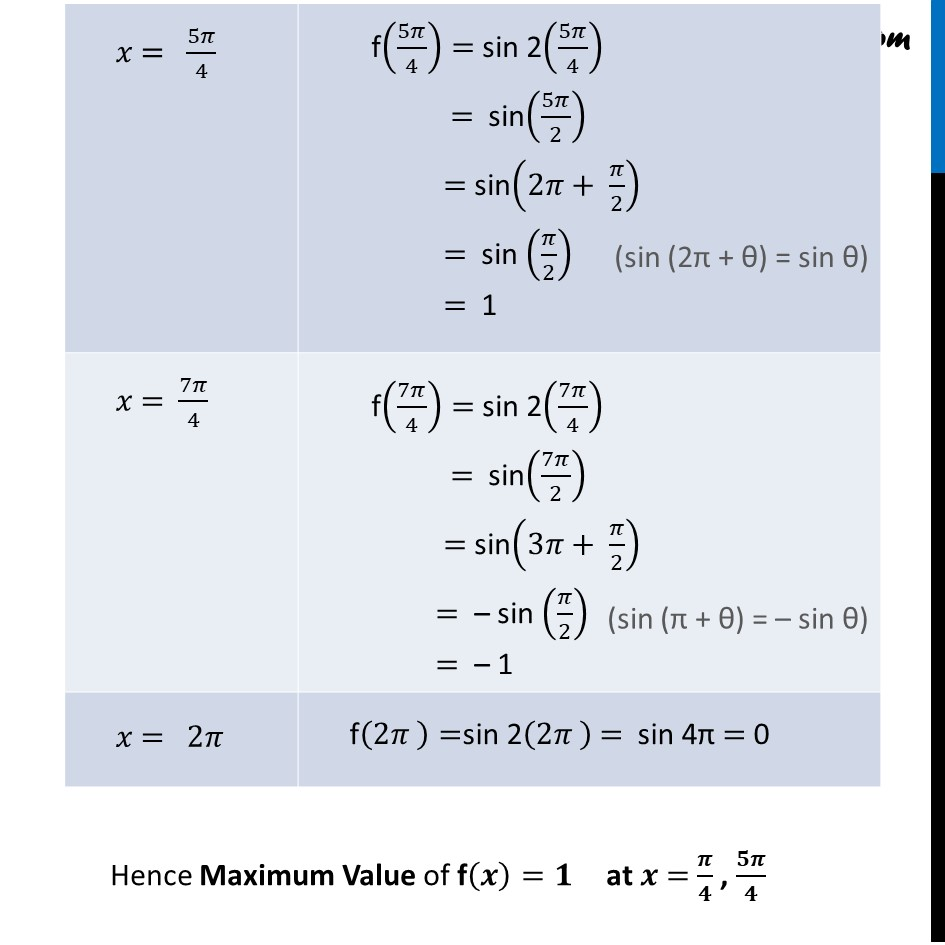Now learn Economics at Teachoo for Class 12

### Transcript

Ex 6.5, 8 At what points in the interval [0, 2π ], does the function sin 2𝑥 attain its maximum value? Let f(𝑥)=sin⁡2𝑥, 𝑥 ∈ [0 , 2𝜋] Finding f’(𝒙) f’(𝑥)=𝑑(sin⁡2𝑥 )/𝑑𝑥 f’(𝑥)=2 cos⁡2𝑥 Putting f’(𝒙)=𝟎 2cos 2𝑥=0 cos 2𝑥=0 cos 2𝑥=cos⁡〖𝜋/2〗 If cos 𝑥=cos⁡𝑦 Then general solution of 𝑥=2𝑛𝜋±𝑦 2𝑥=2𝑛𝜋+𝜋/2 𝑥=(2𝑛+1) 𝜋/4 Putting n = 0 𝑥=(0+1) 𝜋/4= 𝜋/4 Putting n = 1 𝑥=(2+1) 𝜋/4= 3𝜋/4 Putting n = 2 𝑥=(2(2)+1) 𝜋/4= 5𝜋/4 Putting n = 3 𝑥=(2(3)+1) 𝜋/4= 7𝜋/4 Putting n = 4 𝑥=(2(4)+1) 𝜋/4= 9𝜋/4 Putting n = 5 𝑥=(2(5)+1) 𝜋/4 =11𝜋/4>2𝜋 Since 𝑥 ∈ [0 , 2𝜋] So, Critical Point are 𝑥 = 0 , 𝜋/4 , 3𝜋/4 , 5𝜋/4 , 7𝜋/4 & 2π Finding value of f(x) at critical points i.e. 𝑥 = 0 , 𝜋/4 , 3𝜋/4 , 5𝜋/4 , 7𝜋/4 & 2π